﻿ Delayed Mathieu Equation with Fractional Order Damping: An Approximate Analytical SolutionInternational Journal of Mechanics and Applications

p-ISSN: 2165-9281    e-ISSN: 2165-9303

2013;  3(4): 70-75

doi:10.5923/j.mechanics.20130304.02

Delayed Mathieu Equation with Fractional Order Damping: An Approximate Analytical Solution

J. I. Nwamba

Department of Mathematics, University of Nigeria, Nsukka, 410001, Nigeria

Correspondence to: J. I. Nwamba, Department of Mathematics, University of Nigeria, Nsukka, 410001, Nigeria.
 Email: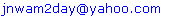Abstract

This paper presents a novel investigation into the dynamics of the time delayed Mathieu equation with fractional order damping given by equation (7). We employed the approximate technique, Adomian Decoposition Method (ADM) in obtaining an analytical result which agreed excellently with the series solution of the above mentioned delay differential equation (DDE) also obtained in this paper. We considered some insightful examples too. It was observed that the ADM gives a solution that is more compact and converges faster when compared to the series solution obtained. Moreover the ADM does not involve tedious calculations and is easier to handle compared to the series method which may become demanding at some point especially if nonlinearity is introduced.

Keywords: Adomian Decomposition Method (ADM), Delay Differential Equation, Fractional damping, Parametric excitation

Cite this paper: J. I. Nwamba, Delayed Mathieu Equation with Fractional Order Damping: An Approximate Analytical Solution, International Journal of Mechanics and Applications, Vol. 3 No. 4, 2013, pp. 70-75. doi: 10.5923/j.mechanics.20130304.02.

1. Introduction

The linear differential equation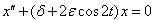(1)
is called Mathieu’s equation. It was first introduced by Mathieu in when he determined the vibration modes of a stretched membrane having an elliptical clamped boundary. Since its introduction, extensive studies have been conducted on/with (1), these studies have revealed the importance of the equation (1) and many of its variation in nonlinear dynamics of real physical systems of engineering and other areas. Reference presented a survey of some of the nonlinear variations of (1). As was noted in, Eq. (1) commonly occurs in nonlinear vibration problems in two different ways. These ways were properly described in. For specified values of the parameters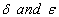, it was noted in[3, 4] that either all solutions of Eq. (1) are bounded (stable) or an unbounded (unstable) solution exists. The existence of stable and unstable solutions to the un-damped Eq. (1) and damped Eq. (2) Mathieu’s equation is attributed to the parametric forcing introduced into the system. Reference investigated the nature and stability of patterns arising from parametric square-wave forcing of an inviscid fluid layer of infinite depth. In, the linear damped Mathieu equation which was derived from the nonlinear PDE’s of the Zhang-Vinals model of a fluid surface under small perturbations, was employed. Reference investigated the
delayed and damped Mathieu’s equation defined by Eq. (3) and determined stability chart connecting the Strut-Ince chart of the damped Mathieu equation (2) and the Hsu-Bhah-Vysnegradskii chart of the autonomous second order delay differential equation. The dynamics of the undamped and delayed Mathieu equation with a cubic nonlinearity Eq. (6) was also investigated by using the method of averaging(2)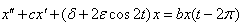(3)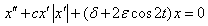(4)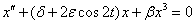(5)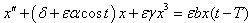(6)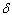is the transient curve,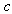is the damping coefficient,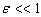is a small parameter and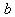is the delay coefficient.
The quadratically damped Mathieu equation defined by (4), happens to be satisfied by the dynamics of towed array lifting devices (LFDs) as shown in. This LFDs help to maintain the position of a tow line being dragged behind. In, the dynamical features of the system (4) was exploited to obtain a control strategy for maintaining the LFD in the desired state. A detailed analysis of Mathieu’s equation especially that of stability can be found in. Furthermore, Mathieu’s equation has wide applications in Boundary Value Problems (BVPs) and recently it has been found out that Mathieu’s equation is practically applied in the following areas: vibrations in an elliptic drum, the inverted pendulum, the radio frequency quadrupole, frequency modulation, stability of a floating body, alternating gradient focusing, the Paul trap for charged particles, and the mirror trap for neutral particles. In all these extensive studies, we note that the fractional aspect of Mathieu’s equation is not left out, see[9,10].

2. The Delayed and Fractional Order Damped Mathieu Equation

Fractional calculus and fractional order differential equations obviously has wide applications in areas like control theory, diffusion, heat conduction, viscoelasticity and electromagnetics. References[9, 10] cites numerous works where such applications were discussed. Many fractional differential equations have been extensively discussed and treated recently. We can find a detailed list of such equations in. In this work, we shall not go into the theoretical and formal basis underlying fractional calculus, but interested readers can see[11-15].
The novel purpose of this work is to, obtain the approximate analytical solution to the delayed and fractional order damped Mathieu equation (7), using two different methods, Adomian Decomposition method (ADM) [10,16-27] whose strong advantage lies in the fact that: (a). it does not transform a given problem into a suitable one for the use of linear theory (b). it does not require the presence of small or large parameter(s) in a given problem (perturbation), and a series method.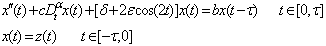(7)
where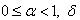is the transient curve conventionally regarded as the frequency of the system squared,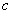is the damping coefficient,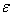is the amplitude of the parametric resonance,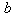is the delay amplitude and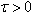is the time delay, and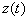is a given function. We note that when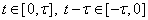, thus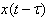may be taken as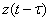on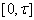.
Then for the interval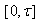, Eq. (7) becomes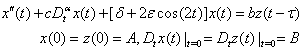(8)
We remark that from idea of the method of steps[28-30], the problem Eq. (8) defined on the interval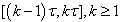, may be solved by successively replacing the R. H. S of Eq. (8) with the solution obtained from the previous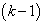equation. Eq. (8) is for the case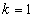.
Following, we define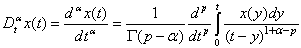(9)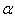, the order of the operation is a positive real number and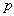is an integer that satisfies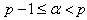.
Setting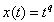in Eq. (9) and performing the resulting integration as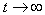, we easily obtain the important formula(10)
We note here that a special case of Eq. (7) where,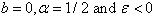was discussed in using the ADM and series method. Also the comprehensive stability analysis of the special case of Eq. (7) where,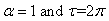which gives rise to Eq. (3) was discussed in.

In this section, we employ the ADM whose basic idea can be found in many literatures, see[10, 16-27], in solving Eq. (8). We rewrite Eq. (8) in the form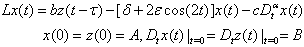(11)
where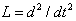is an easily invertible linear operator. In view of the ADM, we write Eq. (11) as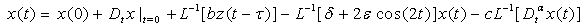(12)
with. Employing the initial conditions given in Eq. (8), Eq. (12) becomes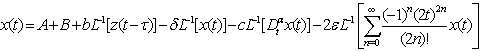(13)
According to the ADM, we can represent the solution Eq. (13) in a series form as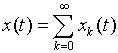(14)
Substituting Eq. (14) into Eq. (13), we obtain the following recurrence scheme for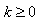: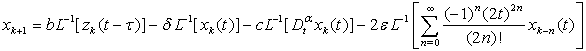(15)
with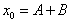. In the light of Eq. (12) and aided by the following relation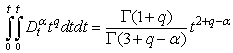(16)
the first few components of the solution can easily be obtained thus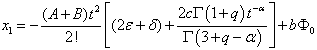(17)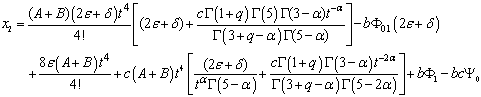(18)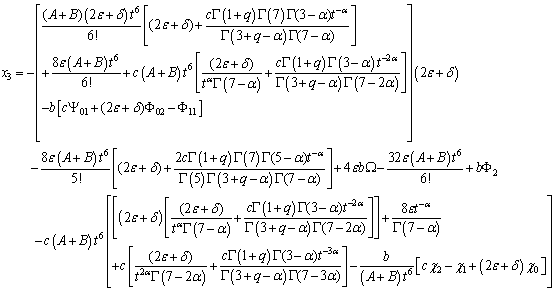(19)
where,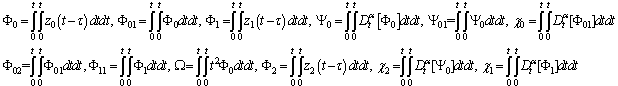Proceeding in the same manner, one may obtain the other higher components of the solution. We remark here that the convergence of solutions obtained through ADM has been meticulously proved in[31-32].

4. Application of a Series Method

We may seek for the solution of Eq. (8) in the form of the fractional power series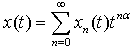(20)
where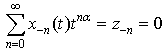(21)
Substituting Eq. (20) into Eq. (8), we have that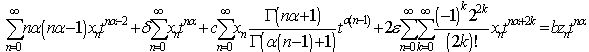(22)
where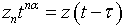. Note that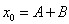, equating the powers of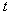in Eq. (22) we can easily obtain the components of the solution for the given parameters.

5. Discussion

In the case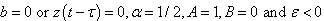, we obtain from Eq. (8)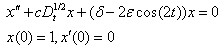(23)
As mentioned above, Eq. (23) was treated in, we note that the same results obtained in Eqs. (13) and (17) of for the ADM and series method respectively were also obtained with the help of Eqs. (17, 18, 19) and (22) in this paper by setting the parameters above equal to their corresponding values.
For the case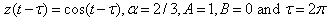, we obtain from Eq. (8)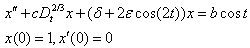(24)
it follows that from Eqs. (17, 18, 19) that for the ADM,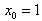and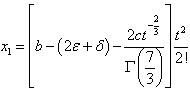(25)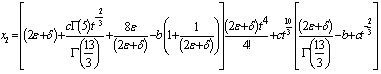(26)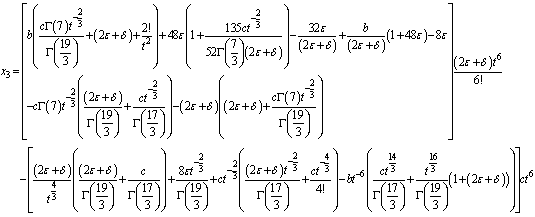(27)
Using Eq. (22) we obtain for the series method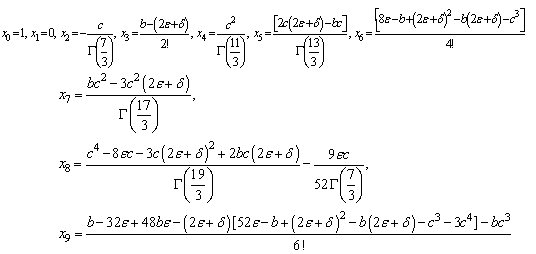(28)
Eq. (28), when combined together gives a series which is exactly the same series obtained by combining Eqs. (25-27).
Furthermore, if we set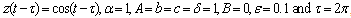, we obtain from Eq. (8)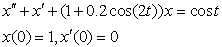(29)
Then it follows from (17), (18), (19) and (22) that the solution to (29) using both the series method and the Adomian decomposition method is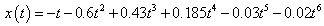(30)
And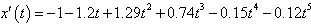(31)
Figure 1 exhibits the behavior of the displacement for (29) employing (30). Figure 2 displays the phase portrait using (30) and (31).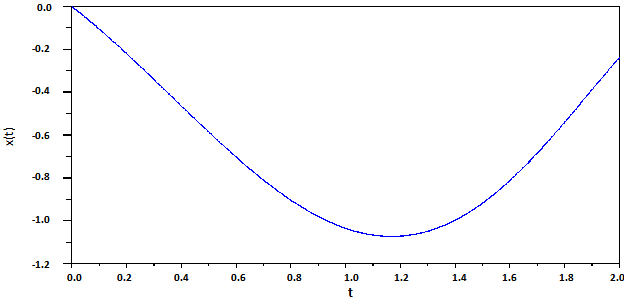Figure 1. Behavior of equation (30)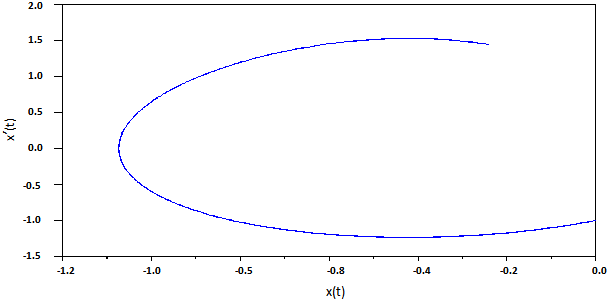Figure 2. Behavior of equation (31)

6. Conclusions

An approximate analytic solution to the delayed Mathieu equation with fractional damping of arbitrary order was obtained in this paper using two different methods viz: Adomian Decomposition method (ADM) and a series method. It was shown that the ADM gives an accurate solution as it was compared to the series solution obtained. Solution to special cases were also considered, especially that treated in. Once more the Adomian decomposition method has been proven to be a powerful tool in nonlinear analysis as it is obvious that accurate analytical solutions to delay differential equations especially fractionally damped ones is very difficult to obtain. Further works in this area can extend its scope to the effect of nonlinearity and forcing on the fractionally damped Mathieu equation.